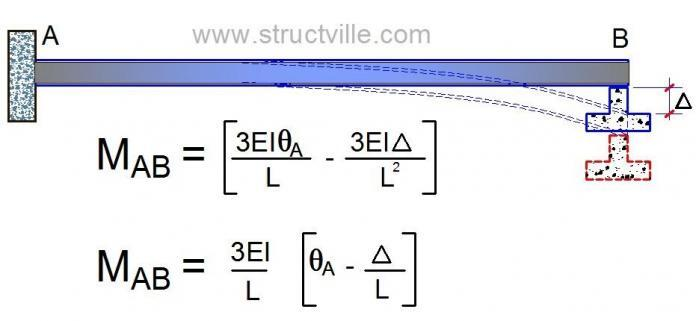# Sinking of Supports of Propped Cantilever BeamsSettlement of foundations can occur due to  loads from the superstructure of buildings. Geotechnical engineers are usually interested in seeing that the settlements are uniform and limited to a certain depth. In some cases however, it is anticipated that differential settlement may occur.

The geotechnical engineer has a responsibility of determining the magnitude of the settlement, while the structural engineer investigates the effect of the settlement on the structural members. If the structure is statically determinate, internal stresses are not induced due to differential settlement. However,  if it is statically indeterminate, then internal stresses due to the settlement are induced. This is usually treated as a special load case in design of structures, and this is what this article explores.

Example
Let us consider a 6m cantilever that is supported on a roller at the free end. The roller support undergoes a vertical settlement of 25mm (downwards). We are to determine the additional bending moment at the fixed support due to the settlement. We will approach this problem using force and stiffness methods.

Solution
(a) By force method
A propped cantilever (roller support) is indeterminate to the 1st order. The adopted basic system for the structure is a simple 6m cantilever. We will now replace the redundant vertical reaction with a unit load, and the resulting bending moment diagram is as shown below.

The appropriate cannonical equation is given by;
δ11X1 + δ1∆ = 0

Where;
δ11 = Deformation at point 1 (free end) due to unit load at point 1
δ1∆ = Deformation at point 1, due to settlement of Support = – EI(∆.S)

δ11 = (1/3) × 6 × 6 × 6 = 72

On the other hand;
δ1∆ =  – EI(∆.S) = -22500 (1 × -0.025) = 562.5

Substituting into the canonical equation and solving;
72X1 + 562.5 = 0
On solving;
X1 = – 7.8125 kN (downward reaction)

With this reaction now known, the moment at support A due to the sinking of support;
MA = – 7.8125 × 6 = -46.875 kNm (hogging moment)

(b) By stiffness method
With support A fully fixed and support B simply supported, the general equation for bending moment at support A due to external load and settlement of support is given by;

MAB = [(3EIθA/L) – (3EI∆/L2)] + FAB

Where FAB is the fixed end moment due to externally applied load = 0
Also θA = 0 since support A is fully fixed.
∆ = 25mm = -0.025m (negative due to downward settlement).

MAB = [((3 × 22500)/6) × (-0.025/6)] = -46.875 kNm

Now, we obtained the answer above expressly because of very known facts. Since the bending moment at B zero, we may not pass through the stress of obtaining the rotation at support B. However for the sake of knowledge, let us apply a more general method which a lot of people might be more familiar with. Here, we will obtain the rotation (slope) at support B, and use it to obtain the bending moment at support A. We are initially assuming that all supports are fixed in the development of the slope deflection equations.

MAB = EI/L[(4θ+ 2θ– 6∆/L)] + FAB
MBA = EI/L[(2θ+ 4θ– 6∆/L)] + FBA

For equilibrium and compatibility;
MBA = 0

We also know that θ= 0 (fixed support), and there is no external load being considered;

22500/6[4θ– (6 × -0.025)/6] = 0
15000θ+ 93.75 = 0

Substituting the value of θinto the slope deflection equation for  MAB
MAB = EI/L[(2θ]
MAB = 3750[(2 × -0.00625)] = 46.875 kNm

Thank you for reading to the end.

2.Donovan